# Branching ratios | Higgs phenomenology

• A
Summary:
I want to understand why some branching ratios (BRs) are greater than others and discuss some other features of the attached plot. Besides, I want to figure out the Feynman diagrams (FDs) I do not find in the literature.

I will bold the ideas I would like to discuss so that they are easier to identify.
Hi everyone!

I am studying Higgs phenomenology, in particular its BRs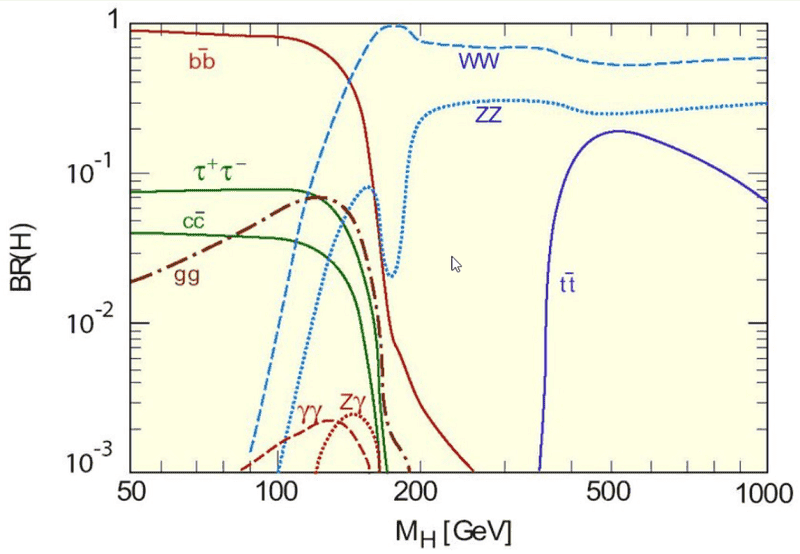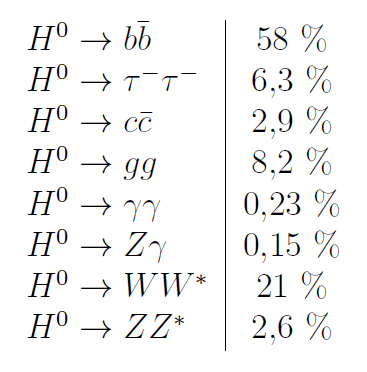My line of reasoning is this: I first had a look at the decays where the Higgs particle couples directly (i.e. without intermediate particles) to the products; those are ##H^0 \to b \bar b, \ H^0 \to \tau \bar \tau## and ##H^0 \to c \bar c \ ## (##H^0 \to t \bar t## is of course not allowed due to conservation of energy; the top quark is about ##t \approx 172 \ GeV## while ##H^0 \approx 125 \ GeV##). Given that the coupling constant is ##m/v##, where the vacuum expectation value is given by ##v \approx 246 \ GeV##, it makes sense to think that the more massive the particle is the stronger it couples to the Higgs particle. Indeed, for those three ratios that argument seems to hold. Do you think it is OK?

Here comes the fun.

Once intermediate particles come into play I do not really understand why some channels have greater BRs than others. Next, let us look at the "massless" channels ##H^0 \to \gamma \gamma, \ H^0 \to gg##. I studied the golden channel ##H^0 \to \gamma \gamma## (Mandl & Shaw, page 446)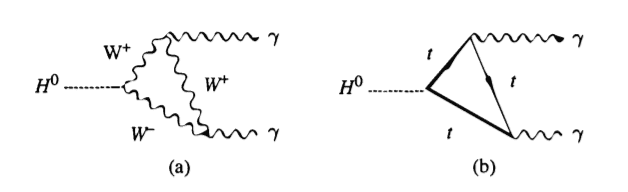OK so W boson and quarks (only the top quark couples significantly to ##H^0## though) are the intermediators.
I struggle to find information in the literature regarding the decay channel ##H^0 \to gg##. I looked up Higgs decay to gluon in Google Scholar and checked the first five but found no (modern i.e. not in terms of ) FD. One could guess and say "well, the gluon is massless as well so we could expect to have the exact same diagrams that for ##H^0 \to \gamma \gamma##" (this is actually the same guess a PF user made back in 2014). I do not think this is right, as the BRs differ quite significantly so I expect the associated FDs to differ as well. Hence

What are the FDs associated to ##H^0 \to gg## and why does its BR differ that significantly with ##H^0 \to \gamma \gamma## (despite both products being massless)?

Regarding FDs, I have the same issue with the channel ##H^0 \to Z \gamma##: I do not find them in the literature.

Finally, let me comment on the last two channels: ##H^0 \to W W^*## and ##H^0 \to Z Z^*##. I have studied they have essentially the same FD (b and c below)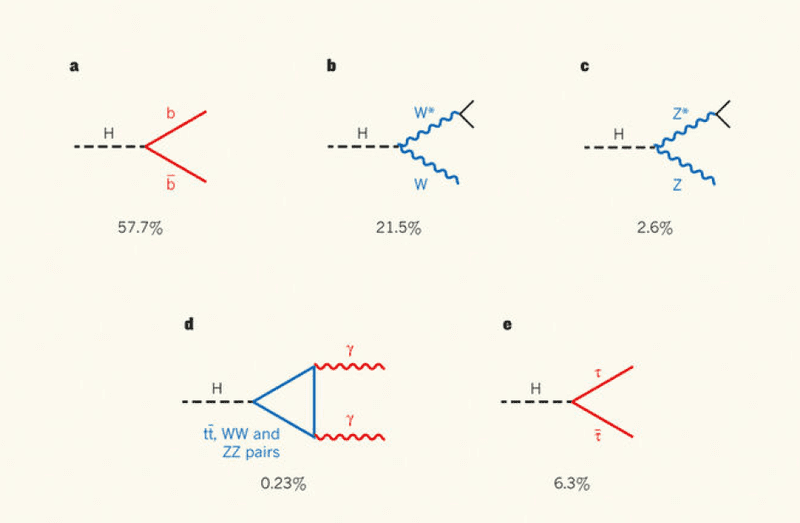My argument to explain their difference in BRs is based on their coupling constants: we know that the Higgs particle couples to W and Z bosons as follows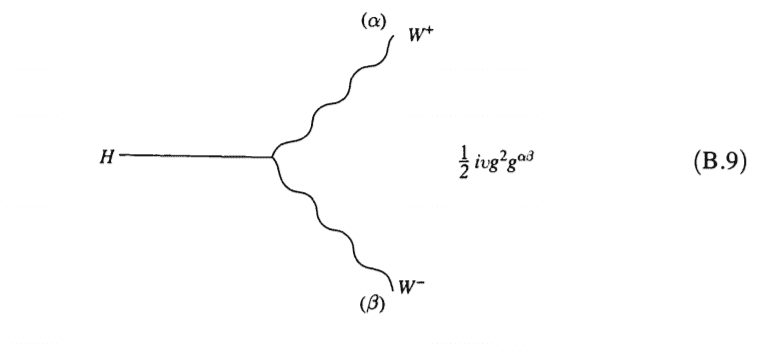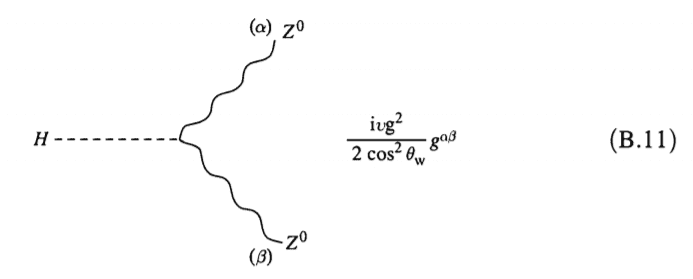We also know that ##M_W^2 = \frac{g^2v^2}{4}## and ##M_Z^2 = \frac{g^2v^2}{4 \cos \theta_W}## so we deduce that the ##HWW## coupling goes as ##M^2_W/v## whereas the ##HZZ## coupling goes as ##M^2_Z/v##. Hence this reasoning leads to think that we should expect a greater BR for Z.

This is wrong and by far... How to explain their BR then?

Last comment: in the plot we observe a sudden minimum in ZZ... why?

I appreciate to discuss with you all

Thank you!•ohwilleke

Orodruin
Staff Emeritus
Homework Helper
Gold Member
Summary:: I want to understand why some branching ratios (BRs) are greater than others and discuss some other features of the attached plot. Besides, I want to figure out the Feynman diagrams (FDs) I do not find in the literature.

I will bold the ideas I would like to discuss so that they are easier to identify.

I do not think this is right, as the BRs differ quite significantly so I expect the associated FDs to differ as well.
Even if the diagrams are similar, the gg amplitudes come with additional colour factors.

•ohwilleke
Hi, these are interesting questions.

1) H to gg
-FD ?
Same with gg to H production. Gluon radiations through quark triangle.
-Why BR(H to gamma gamma) << BR(H to gg)?
There is another factor, the coupling constant.
You can compare alpha_s (~ 0.1) and alpha_em (~1/137) to understand difference between two BRs.

2) FD of H to Zgamma
It is same with H to gamma gamma. Replace one gamma to Z.

3) BR(H to WW) vs BR(H to ZZ*)
H to W-W+ channel has factor of two bigger BR compared to ZZ since W- and W+ are not identical particles.
(JHEP03(2018)110)

4) Sudden minimum of BR(H to ZZ*)
If mH < 2mW, there is suppression coming from virtuality of W* and Z*.
If 2mW < mH < 2mZ, H to WW channel gets enhancement from on-shell effect.
Because of this enhancement, BR(H to ZZ) shows sudden minimum.
After mH > 2mZ, BR(H to ZZ) and BR(H to WW) show almost flat values until mH = 2mtop.

•ohwilleke, JD_PM and mfb
Even if the diagrams are similar, the gg amplitudes come with additional colour factors.

Thank you.

Hi, these are interesting questions.

1) H to gg
-FD ?
Same with gg to H production. Gluon radiations through quark triangle.
-Why BR(H to gamma gamma) << BR(H to gg)?
There is another factor, the coupling constant.
You can compare alpha_s (~ 0.1) and alpha_em (~1/137) to understand difference between two BRs.

Oh, I see! At low energies, on expects to have a small EM fine structure constant ##\alpha \approx \frac{1}{137}## and a significant (so no perturbation techniques can be used) QCD constant.##g_s \approx 0.1##. I am just wondering why the latter value is not that well-known (I guess that the reason is due to not being really useful, as one cannot use perturbation theory?).

3) BR(H to WW) vs BR(H to ZZ*)
H to W-W+ channel has factor of two bigger BR compared to ZZ since W- and W+ are not identical particles.
(JHEP03(2018)110)

Could you please elaborate on this? Or alternatively point me at the section of the paper in which one can understand why there is this difference in the BRs? (I have read the abstract and dived through it via the key word "decay" but I did not find the explanation). Besides I found the same question in PSE but the answer does not really help me to get the point ...

4) Sudden minimum of BR(H to ZZ*)
If mH < 2mW, there is suppression coming from virtuality of W* and Z*.
If 2mW < mH < 2mZ, H to WW channel gets enhancement from on-shell effect.
Because of this enhancement, BR(H to ZZ) shows sudden minimum.
After mH > 2mZ, BR(H to ZZ) and BR(H to WW) show almost flat values until mH = 2mtop.

•ohwilleke
mfb
Mentor
It's more instructive to look at the partial widths instead of branching fractions. Branching fractions depend on all other decay modes, partial widths do not.
Using the WW bump as an example: There is nothing special happening to ZZ there. It's just the WW partial width becoming so much larger that it pushes down all other branching fractions - not just ZZ. ZZ would become "competitive" again at masses where the Higgs can decay to two on-shell Z.

•JD_PM, vanhees71 and ohwilleke
ohwilleke
Gold Member
Somewhat related question. I've seen statements that the diphoton branching fraction is maximized at about 125 GeV. Does anyone know if there's a source pinning down the Higgs mass that maximizes the diphoton branching fraction to greater precision than that (e.g. is it actually 125.23 GeV?)?

mfb
Mentor
You could try to fit tables like this one. 125.6-125.7 as best fit.

•dextercioby and ohwilleke
Staff Emeritus
Before we get started, I think the hypothesis you have expressed elsewhere that there is some sort of significance to the Higgs to gamma gamma branching fraction being close to the maximum is about as likely to be correct as the idea that there is some sort of significance to the Higgs mass being the country code of Switzerland, where it was discovered, in Base 31. (Which is itself the country code for Holland, where some of the LHC magnets were made.)

Second, you need to consider errors and not just central values in @mfb's table. The maximum of the H→γγ branching fraction is 125 ± 5 GeV or so. So at 95% CL it could be anywhere between 115 and 135.

•ohwilleke and mfb
mfb
Mentor
The uncertainties in the individual rows should be highly correlated. Probably close to 100%.

•ohwilleke
Staff Emeritus
The uncertainties in the columns are correlated, because it's the same calculation. The uncertainties in the rows are anticorrelated, because the branching fractions need to sum to 1.

My estimate comes from the bbar branching fraction which has some uncertainties depending on the b-quark mass and higher order corrections, which is a) large and b) changing with Higgs mass. Move it around and the point where gmma-gamma is maximal moves too. (Oppositely - shift bbar to the left and gamma gamma moves to the right)

Last edited:
•ohwilleke
ohwilleke
Gold Member
Before we get started, I think the hypothesis you have expressed elsewhere that there is some sort of significance to the Higgs to gamma gamma branching fraction being close to the maximum is about as likely to be correct as the idea that there is some sort of significance to the Higgs mass being the country code of Switzerland, where it was discovered, in Base 31. (Which is itself the country code for Holland, where some of the LHC magnets were made.)

Second, you need to consider errors and not just central values in @mfb's table. The maximum of the H→γγ branching fraction is 125 ± 5 GeV or so. So at 95% CL it could be anywhere between 115 and 135.
Could be (wasn't my idea in the first place, I've just rounded up a variety of non-mutually consistent ideas I'd seen other published works express).

But I'm nonetheless curious anyway, even if it is just a pure coincidence or trivial pursuit class data point.

mfb
Mentor
The uncertainties in the columns are correlated, because it's the same calculation. The uncertainties in the rows are anticorrelated, because the branching fractions need to sum to 1.
I meant the individual rows within the diphoton column, i.e. what you call "in the columns".
(wasn't my idea in the first place, I've just rounded up a variety of non-mutually consistent ideas I'd seen other published works express)
Who published the idea that the highest diphoton branching fraction would be relevant?

Staff Emeritus
•